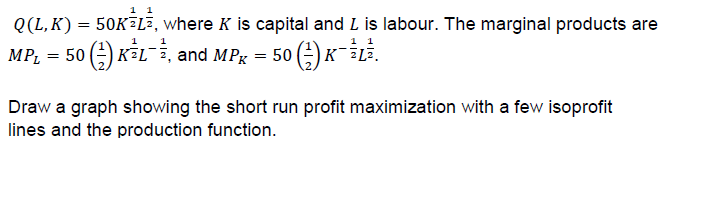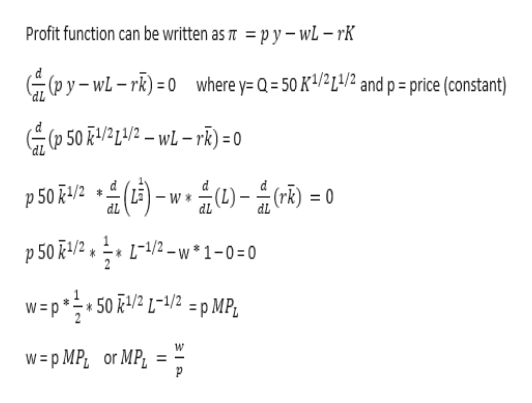# 1 1Q(L, K)50KELE, where K is capital and L is labour. The marginal products are1 111к -LE.KFL, and MPgMPL5050Draw a graph showing the short run profit maximization with a few isoprofitlines and the production function.

Question
9 viewshelp_outlineImage Transcriptionclose1 1 Q(L, K) 50KELE, where K is capital and L is labour. The marginal products are 1 1 1 1 к -LE. KFL, and MPg MPL 50 50 Draw a graph showing the short run profit maximization with a few isoprofit lines and the production function. fullscreen
check_circle

Step 1

Let assume that the cost of capital is r and cost of labor (wages) is w

To obtain maximization condition for any function, it must be differentiated wrt to a variable and set equal to zero.

In the short-run capital is constant, so profit function will be differentiated wrt to L and set equal to zero. Let capital be fixed at k-bar.

Profit function can be written as shown below.help_outlineImage TranscriptioncloseProfit function can be written as 7 = py-wL - rK pywL-r) 0 where y= Q = 50 K4/21/2 and p = price (constant) dL (p 501/21/2-wL-rk)=0 d (ri p 50R4/2 p 50 /2 L-1/2-w 1-0 0 w=p50/2 L1/2 = p MP, W w=p MP, or MPL fullscreen
Step 2

To graphically represent the profit maximization, let’s say that the maximum profit is equal to an arbitrary number pie-bar

Step 3

The graph with isoprofit line wil...

### Want to see the full answer?

See Solution

#### Want to see this answer and more?

Solutions are written by subject experts who are available 24/7. Questions are typically answered within 1 hour.*

See Solution
*Response times may vary by subject and question.
Tagged in